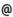## "Babes-Bolyai" University of Cluj-Napoca Faculty of Mathematics and Computer Science

 Numerical methods in Fluid Dynamics
 Code Semes-ter Hours: C+S+L Credits Type Section MM271 2 2+2+0 9 compulsory Modele matematice în Mecanică şi Astronomie
 Teaching Staff in Charge
 Prof. PETRILA Titus, Ph.D., tpetrilacs.ubbcluj.ro
 Aims The course presents advanced topics in Fluid Dynamics with a special accent on analitical and numerical approximation methods. Subsonic, supersonic and transonic motions are analized through examples, and in each case several approximation methods are discussed.
 Content Deformable Continuum's Equations. The case of Inviscid Fluid and the case of Viscous Fluid. Numerical methods for second order Partial Differential Equations. Stability, consistence and convergence for Finite Difference Schemes. Finite Element methods. Irrotational Flows of the Incompressible Inviscid Fluids in two Dimensional Case. Conformal Mapping. Principles of the Profiles Theory. Notions on the Hodograph Method. Approximation method for Conformal Mapping. Panels method (Sources or Vortices) for Two Dimensional Flows past a profile. Theory of Thin Airfoils. The Steady Irrotational Flows produced by the Motion of an Obstacle in an Ideal Fluid. Boundary Element Methods and CVBEM applied in profile theory. Barotrop Compressible Fluid Flows. Stechen's equations. Analytical methods for the approximation of the corresponding nonlinear system. Theory of Linearization's principle. The Glauert-Prandtl' rule and Ackeret' rule. Finite Difference schemes. Diffusion Equation. Viscous incompressible fluids flow. Adimensional variables. Burgers' equations, Analytical solution, solving the nonlinearity through adequate finite difference schemes. Flows with large Reynolds numbers. Boundary Layer equation. Flows with small Reynolds numbers. Stokes system. Numerical methods based on Integral equations. Navier-Stokes equations. Approach with the finite element method.
 References 1. TITUS PETRILA, DAMIAN TRIF, Metode numerice si computationale in mecanica fluidelor, Edit. Digital Data, Cluj-Napoca, 2002 2. TITUS PETRILA, CALIN GHEORGHIU Metode element finit si aplicatii, Editura Academiei Române, 1987 3. CAIUS IACOB, Introduction mathematique a la mecanique des fluides. editura Academiei Române si Editions Gauthier-Villars, 1959. 4. LAZAR DRAGOS, Principiile mecanicii mediilor continue, Editura Tehnica, Bucuresti, 1981 5. DANIEL EUVRARD, Resolution numerique des equations aux derivees partielles, editions Masson, Paris, 1994 6. CHUEN YEN CHOW, An Introduction to Computational Fluid Dynamics, John Wiley & Sons, NY, 1980 7. D. J. ACHESON, Elementary Fluid Dynamics, Clarendon Press, 1992 8. H. DUMONTET, GEORGES DUVAUT,ETC Exercices de mecanique des millieux continus, Editions Masson, Paris, 1994 9. TITUS PETRILA, Lectii de mecanica mediilor continue, lito UBB, 1980 10. PAUL GERMAIN, Mecanique des millieux continus, Editions Masson, Paris, 1962 11. TITUS PETRILA, DAMIAN TRIF, Basics of Fluid Mechanics and Introduction to Computational Fluid Dynamics, Springer USA, 2005
 Assessment Exam.